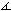E. W. Dijkstra's Proof of the Pythagorean Theorem

The essence of Dijkstra's approach to the old theorem is less an elegant proof than a novel formulation. Dijkstra's formulation serves a bridge to the Law of Cosines but even more curiously it focuses on the comparative properties of all angles of a triangle instead of a customary consideration of a single angle, which is required to be right for the Pythagorean theorem or anything else for the Law of Cosines or generalizations.

If, in a ΔABC, angles α, β, γ lie opposite the sides of length a, b, c, then

 sign(α + β - γ) = sign(a² + b² - c²),

where sign(t) is the signum function.

This applet requires Sun's Java VM 2 which your browser may perceive as a popup. Which it is not. If you want to see the applet work, visit Sun's website at https://www.java.com/en/download/index.jsp, download and install Java VM and enjoy the applet.

 What if applet does not run?

Dijkstra constructs two additional lines CL and CN so thatBCN =CAB andACL =CBA

which makes triangles ABC, ACL and BCN similar and angles ALC and BNC equal. At the vertex C we now find and may compare all three angles α, β, γ of ΔABC. Dijkstra's original diagram only deals with the case where α + β - γ < 0 which means that the triangles ACL and BCN do not cover ΔABC completely implying the inequality

 Area(ACL) + Area(BCN) < Area(ABC).

In view of α + β + γ = 180°, α + β - γ = 0 entails γ = 90° and also α + β = 90° so that, in this case, CL and CN coincide and the diagram becomes that of Proof #6. Thus α + β - γ = 0 implies Area(ACL) + Area(BCN) = Area(ABC).

Finally, wherever α + β - γ > 0, the two triangle ACL and BCN overlap. It is even possible just for one of them to cover ΔABC completely. In any event, if α + β - γ > 0 we have

 Area(ACL) + Area(BCN) > Area(ABC).

So in all cases,

 sign(α + β - γ) = Sign(Area(ACL) + Area(BCN) - Area(ABC)).

To finish the proof, one only needs to observe that the areas of similar triangles are in the same proportion as the squares of any of their corresponding elements. In particular, picking up the sides opposite the angle equal to γ

 Area(ACL) / a² = Area(BCN) / b² = Area(ABC) / c²,

or

 Area(ACL) = ka², Area(BCN) = kb², Area(ABC) = kc²

for some positive k. Which leads directly to Dijkstra's claim:

 sign(α + β - γ) = sign(a² + b² - c²).Intuitively clear as Dijkstra's proof may be, not everyone finds it simple enough. I have received the following from Mr. Guoping Zeng, Ph.D. in Math, Sr. Software Engineer, Adjunct Professor, UT Dallas:

The geometric proof of Dijkstra's generalization of the Pythagorean theorem is too complicated. Moreover, the figure for the case is not easy to draw considering many cases: β = γ, α = β = γ, α > γ, etc.

Indeed, the following algebraic proof by means of the Law of Cosines is much simpler and nicer.

Algebraic Proof (G. Zeng): Since α + β + γ = π, we have α + β - γ = π - 2γ. Taking the sign function on both sides, we have

 (1) sign(α + β - γ) = sign(π - 2γ) = sign(π/2 - γ).

By the Law of cosines, c² = a² + b² - 2ab cosγ. Re-arranging the terms yields

 (2) a² + b² - c² = 2ab cosγ.

Taking the sign function on both sides, we have

 (3) sign(a² + b² - c²) = sign(2ab cosγ) = sign(cos γ).

Since cos γ is positive, zero and negative when γ < π/2, γ = π/2, and γ > π/2, respectively, we have

 (4) sign(cos γ) = sign(π/2 - γ).

Substituting (4) into (3) yields

 (5) sign(a² + b² - c²) = sign(2ab cosγ) = sign(π/2 - γ).

Comparing (5) and (1) yields

 (6) sign(a² + b² - c²) = sign(α + β - γ).

Q.E.D.

As often is done in mathematics, see for example a discussion in [D. Ruelle, The Mathematician's Brain], mathematicians help their thinking and interpersonal communication by relying on unique concepts that incorporate and, thus, hide the complexity of the constructions based on first principles. Seldom mathematicians derive their results directly from some fundamental axioms. Instead, they use already proven theorems and suitable definitions. For example, the cosine function embodies the idea of similarity, as characterized by shape properties of triangles, and sign switching depending on the size of angle γ implicit in Euclid II.12 and II.13. The Law of Cosines, too, admits multiple proofs that do not rely on the Pythagorean theorem so that Dr. Zeng's proof does not include any circularity and constitutes a valid derivation of Dijkstra's identity.